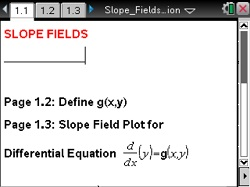# Activities

••• ##### Subject Area

• Math: Calculus: Antiderivatives and Slope Fields

• ##### Author9-12

45 Minutes

• ##### Device
• TI-Nspire™
• TI-Nspire™ CAS
•TI-Nspire™ CX/CX II
•TI-Nspire™ CX CAS/CX II CAS
• TI-Nspire™ Navigator™
• TI-Nspire™ Apps for iPad®
• ##### Software

TI-Nspire™
TI-Nspire™ CAS

3.6

• ##### Report an Issue

Slope Fields#### Activity Overview

Use a visual representation of the family of solutions to a differential equation.

#### Objectives

• Use a visual representation of the family of solutions to a differential equation

#### About the Lesson

The Slope_Fields.tns TI-Nspire document provides a graphical tool for visualizing antiderivatives and, more generally, solutions to differential equations. Slope fields are motivated by the idea of “local linearity”—a differentiable function behaves very much like a linear function on small intervals. Using that idea, if you know the value of the derivative of a function at a single point, then you can approximate a small portion of its graph with a straight line segment centered at that point, having the required slope. If you know the derivative value at every point, then you could choose a large sample of points (for example, a rectangular lattice of grid points) and plot a small slope segment at each one, creating a slope “field” (much like a direction field for vector plots). The result provides a powerful way to visualize solution curves (graphs of solution functions), even for differential equations that would defy paper-and-pencil techniques or the use of a computer algebra system.